Next: 3.1.5 The Insulator Equations Up: 3.1 Sets of Partial Previous: 3.1.3 The Hydrodynamic Transport

## 3.1.4 The Lattice Heat Flow Equation

MINIMOS-NT accounts for self-heating effects in semiconductor devices by solving the lattice heat flow equation self-consistently with the DD or HD transport equations, forming together a system of four, or respectively six, partial differential equations.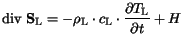(3.14)(3.15)

In (3.14)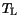denotes the lattice temperature,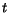is the time variable, and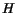is the heat generation term. The coefficients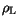,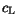, andare the mass density, specific heat, and thermal conductivity of the respective materials.

The model for the heat generation,, depends on the transport model used. In the drift-diffusion caseequals the Joule heat,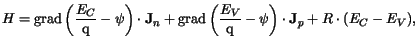(3.16)

whereas in the hydrodynamic case the relaxation terms are used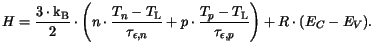(3.17)Next: 3.1.5 The Insulator Equations Up: 3.1 Sets of Partial Previous: 3.1.3 The Hydrodynamic Transport
Vassil Palankovski
2001-02-28